# MCQs on Electric Circuits

##### Page 27 of 63. Go to page 1 2 3 4 5 6 7 8 9 10 11 12 13 14 15 16 17 18 19 20 21 22 23 24 25 26 27 28 29 30 31 32 33 34 35 36 37 38 39 40 41 42 43 44 45 46 47 48 49 50 51 52 53 54 55 56 57 58 59 60 61 62 63
01․ A capacitor is connected to supply with switch and the switch is connected between capacitor and supply. Initially switch is open at time zero, and then switch is closed. Then how capacitor behaves at time t = 0+?
short circuit.
open circuit.
dielectric losses decreases.
dielectric losses increases.

In case of a capacitor, voltage across it does not change instantaneously. If an uncharged capacitor is connected to an energy source, at the time of switching, the capacitor will behave like a short circuit.

02․ Which of the followings is the expression for energy stored in a capacitor?
Cv.
C dv / dt.
C / v.
(1 / 2) Cv2.

The instantaneous power in the capacitor is given by p = iv.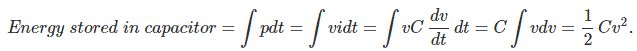03․ A parallel plate capacitor has a capacitance of C farad. It area of the plates is doubled and the distance between them is half, the capacitance of the capacitor is

A capacitor consists of two parallel places separated by a dielectric material. It the area of the plates is A m2 and the distance between them is d meter, the capacitance C is given by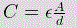Where A is the area of the plates and d is distance between the plates. From the above expression of capacitance it is obvious that, if area is doubled and distance is half the capacitance will become 4 times.

04․ A capacitor carries a charge of 0.3 C at 20 V. Its capacitance is
1.5 F.
0.015 F.
1.5 μF.
15 μF.

The capacitance of a capacitor is expressed as,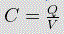Where, C is the capacitance, Q is charge and V is the voltage.

05․ If a capacitors of capacitance 100 μF is connected across a voltage source of 10 V, then what will be the energy stored in that capacitor?
5 × 10 - 3 Joule.
10 × 10 - 3 Joule.
10 × 10 6 Joule.
5 × 10 - 2 Joule.

It a capacitor of capacitance value C have voltage difference V between its parallel plates then the energy stored in the capacitor is expressed as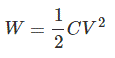06․ When AC flows through a pure capacitance then the current
lags the emf by 90o.
leads the emf by - 90o.
is in phase with emf.

When AC flows through an capacitance, the current leads the emf by 90o.

07․ The capacitive reactance of a capacitor of (1 / 2μF) F at 103 Hz is
10 6 μF.
10- 3 μF.
10 3 μF.
10- 6 μF.

The capacitive reactance can be expressed as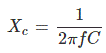08․ A 20 μF capacitor and 200 W, 220 V lamp is connected in series across a 220 V alternating supply. In which frequency of the supply the lamp will glow dimmest?
1000 Hz.
1 Hz.
10 Hz.
100 Hz.

The impedance of capacitor is inversely proportional to its supply frequency. The impedance offered by the capacitor to the circuit is more when supply frequency is less. If impedance is more, current flowing through the circuit (i.e. lamp) is less. Lamp will glow dimmest when minimum current will flow i.e when frequency is minimum.

09․ A 10 μF capacitor and 100 W, 220 V lamp is connected in series across a 220 V alternating supply. In which frequency of the supply the lamp will glow brightest?
1000 Hz.
100 Hz.
10 Hz.
1 Hz.

The impedance of capacitor is inversely proportional to its supply frequency. The impedance offered by the capacitor to the circuit is more when supply frequency is less. If impedance is less, current flowing through the circuit (i.e. lamp) is more. Lamp will glow brightest when maximum current will flow i.e when frequency is maximum.

10․ A capacitor passes a current of 12.6 mA when supplied with 20 V ac with a frequency of 1000 Hz. The capacitance will be
0.001 μF.
0.01 μF.
0.1 μF.
1 μF.

If Vc is the voltage across capacitor, Ic current through the capacitor, f and C are frequency and capacitance then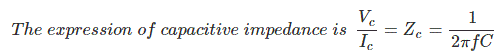Here, Vc = 20 V, Ic = 12.6 mA and f = 1000 Hz.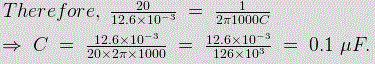<<<2526272829>>>